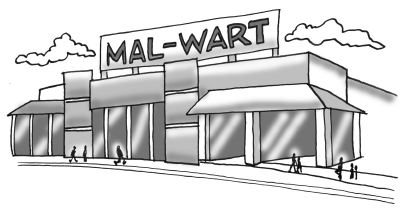### Home > GB8I > Chapter 8 Unit 9 > Lesson INT1: 8.1.3 > Problem8-52

8-52.

After noon, the number of people in Mal-Wart grows steadily until $6\text{:}00$ p.m. If the equation $y = 228 + 58x$ represents the number of people in the store $x$ hours after noon:

1. How many people were in the store at noon?

Find the starting value in the equation.

2. At what rate is the number of shoppers growing?

Find the growth rate in the equation.

3. When were there $402$ shoppers in the store?

Set the equation equal to $402$ and solve for $x$.Use the eTool below to help solve the problem.
Click the link at the right to view full version of the eTool: Int1 8-52 HW eTool.